A Worked Example: Common Tangent Construction and Spinodal Points  (Part B: Computing the Common Tangent)

We can use the ConvexHull to find the common tangent lines; this function is in the Computational Geometry Package.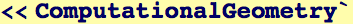First we compute a list of values along the molar free energy curve, then compute those that lie outside the common tangent(s) (i.e., the convex hull). Because the points are given in order, we might as well
sort them on the way back out.  Note, the convex hull program gives the indices of the vertices that are on the hull.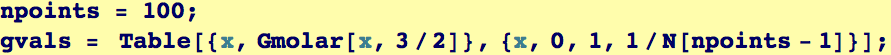We only want the lower convex hull; therefore we add some "fictive" points to the beginning and the end of the data.  The the fictive points add a rectangle to the top of the curve that should be part of the computed convex hull.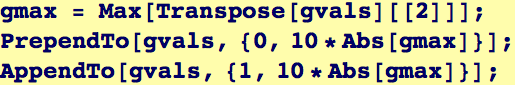After we compute this hull, we shift the hull by one and take off its first and last element. We strip the first and last element from the discrete values of free energy as well.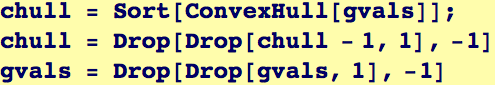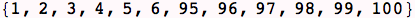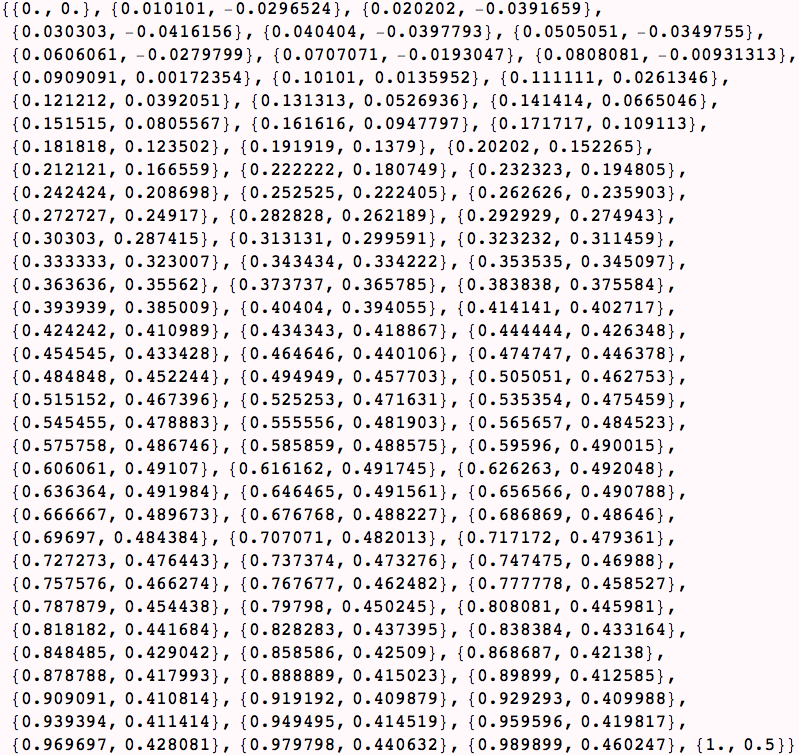The common tangent(s) correspond to gaps in the vertex list of the common tangent. We will use Split to find the set of continous sequences.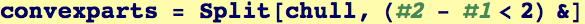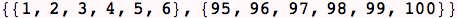Created by Wolfram Mathematica 6.0  (28 August 2007)